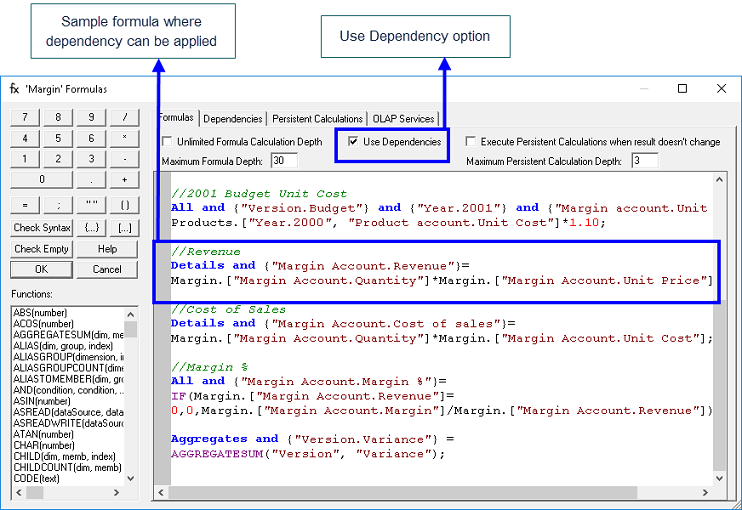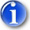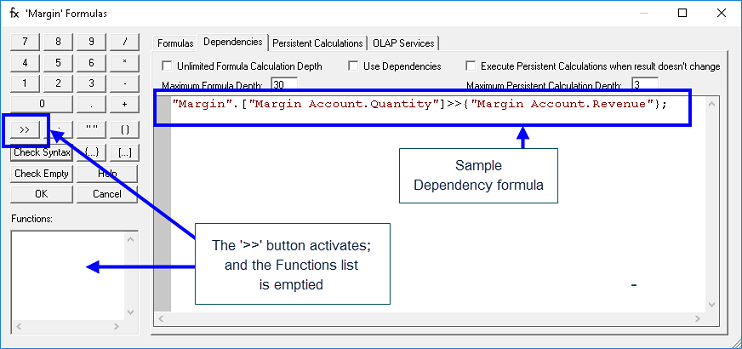DependenciesDependencies are a means to optimize calculations in Cubes that use formulas: the use of Dependencies can yield dramatic calculation speed increases. With Dependencies you can specify precise areas of a Cube so that aggregations will only execute on cells that have Dependencies that refer to non-zero values.

In Cubes that have no formulas, the PowerOLAP aggregation engine does not “mark” a cell for aggregation if its value is zero. (Thus, if Canada, Mexico and U.S. aggregate to North America, then there is no need to include Canada in the North Americaaggregation of, say, Revenue, if Canada’s Revenue value is zero.) When formulas are written for a Cube, this aggregation engine feature is canceled—precisely because a formula may govern the cell, resulting in an ultimate value (i.e, formula-driven value) that is not zero.

The following example illustrates how you can apply knowledge of a model to reduce calculation “cost.” Consider a Cube with a Margin Account dimension whose Members include Revenue, Unit Price and Quantity and where Revenue is governed by the formula: Revenue = Unit Price  X Quantity. Given the fact that a formula exists in the Cube, each cell in a Slice must be examined for the purposes of aggregations. This examination can be costly because the aggregation engine does not skip cells the way it can when formulas are not present. Thus, to optimize the formula calculation, you could make the calculation of aggregations involving Revenues depend on the value of Quantity, whose values can be zero (0) and any other value.

In plain English, you would set up a Dependency relationship argument that states, “When a Quantity value is not zero and therefore will not yield a zero result in the formula governing Revenue cells, put a marker to calculate aggregations for those cells (by implication, do not perform the aggregation on the cells governed by the formula that will yield a zero result because Quantity is zero).” Below are the example formula and its Dependency relationship:

Formula:

 Details and {“Margin Account.Revenue”}=Margin.[“Margin Account.Quantity”]*Margin.[“Margin Account.Unit Price”];

Dependency

 Margin.[“Margin Account.Quantity”]>>{“Margin Account.Revenue”};

[Note that although Details is specified for the calculation of the formula for Revenue on the left-hand side, this is not required on the right-hand side of the Dependency relationship—Dependency relationships are understood to apply to Detail-level cells.]

While the elimination of the examination of Revenue cells for aggregations may seem minor in this simple example, one must remember that there are potentially hundreds of thousands of Revenue cells in our hypothetical PowerOLAP model. In this manner, in complex formula-driven models, Dependencies can provide very large computational savings.

In order to take advantage of Dependency optimization, you will need to perform two steps.

You must inform the calculation engine, using a checkbox—see the following figure—to use Dependencies. (Refer to the succeeding discussion on User Considerations.)

You will want to specify the set of “precedent” non-zero cells on which formulas are executed—i.e., the set of cells for which it must execute aggregations in a dependency relationship.

The set of cells depends on a region in either the same Cube as that of the formula or in a different, external Cube (i.e., in a cross-cube formula). The Dependency relationship is specified in the same Cube in which a formula is defined that can make use of the Dependency.

The Formulas dialog box provides a Dependency editor reached via the Dependencies tab.The Formulas dialog box contains three tabs: Formulas, Dependencies and Persistent Calculations. The Formulas tab enables a text box where, as you have seen, you define formulas in a Cube (the above figure shows an example formula for calculating Revenue, boxed, to which a Dependency might be applied).

The Use Dependencies checkbox (boxed in the figure) determines whether Dependencies are to be used when calculating the aggregations for cells governed by formulas in the Cube.ImportantBy Checking the Use Dependencies checkbox, you have committed the Cube to apply Dependency logic to all saved formulas. Thus, you must consider the ramifications, i.e., both the effect on aggregation calculations if no Dependency relationship is specified and the effect when you do define a Dependency relationship for a formula. Again, see User Considerations topic.

The following figure shows the Dependencies editor, with a defined Dependency relationship:When the Dependencies tab is selected, thebutton from the Formulas tab changes into thebutton. The function list is emptied (i.e., deactivated) since these right-hand side functions cannot be used in Dependencies. The remaining features function as they did when the Formulas tab was active.NotesIn the event that dependencies were inefficiently written this could result in the inability to open the database due to excessive memory usage. In cases like this, it is advised to enable the Open Database in Safe Mode option when opening the database. Refer to the Open a PowerOLAP Database topic under the Working with Slices section of this manual for more information on the Open Database in Safe Mode option.